# Difference between revisions of "Ellipsoid in three-dimensional Euclidean space"

## Definition

This surface type is not unique up to isometry or even up to similarity transformations, but rather, depends upon multiple nonzero parameters$a,b,c$. If we're considering the surface up to rigid isometries, the parameters are unique up to permutations. If we're considering the surface up to similarity transformations, the parameters are unique up to the action of permutations and projective equivalence.

### Implicit and parametric descriptions

Degree of generality Implicit description What the parameters mean Parametric description What the additional parameters mean Comment
Arbitrary Fill this in later This version need not be centered at the origin and need not be oriented parallel to the axes.
Up to rotations$\frac{(x - x_0)^2}{a^2} + \frac{(y - y_0)^2}{b^2} + \frac{(z - z_0)^2}{c^2} = 1$$x = x_0 + a\cos u \sin v, y = y_0 + b \sin u \sin v, z = z_0 + c \cos v$ This version need not be centered at the origin but is oriented parallel to the axes.
Up to rigid motions (rotations, translations, reflections)$\frac{x^2}{a^2} + \frac{y^2}{b^2} + \frac{z^2}{c^2} = 1$$a$,$b$,$c$ are positive numbers that represent the lengths of the three ellipsoidal semi-axes. Note that because we allow rigid motions, we can permute and rearrange to force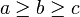$a \ge b \ge c$.$x = a\cos u \sin v, y = b \sin u \sin v, z = c \cos v$ Here,$u$ and$v$ are ellipsoidal equivalents of the angles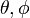$\theta, \phi$ used in spherical polar coordinates. This version is centered at the origin and oriented parallel to the axes.
Up to similarity transformations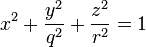$x^2 + \frac{y^2}{q^2} + \frac{z^2}{r^2} = 1$ Here,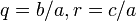$q = b/a, r = c/a$. If we chose$a \ge b \ge c$, we would get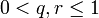$0 < q,r \le 1$. For this version, we can normalize one of the three values$a,b,c$. In the form given here, we chose to normalize$a$.

## Particular cases

All parameter values$a,b,c$ are equal 2-sphere in Euclidean space This has full rotational symmetry about every axis through its center and reflection symmetry about every plane through its center.
Two of the parameter values are equal, and they are both greater than the third oblate spheroid This has full rotational symmetry about the third axis, and can be obtained by taking the surface of revolution obtained from an ellipse in a plane through that axis. The ellipse is being revolved about its minor axis.
Two of the parameter values are equal, and they are both less than the third prolate spheroid This has full rotational symmetry about the third axis, and can be obtained by taking the surface of revolution obtained from an ellipse in a plane through that axis. The ellipse is being revolved about its major axis.

## Fundamental forms and curvatures

For the table below, we consider the parametric description that is valid for$\frac{x^2}{a^2} + \frac{y^2}{b^2} + \frac{z^2}{c^2} = 1$

namely the description: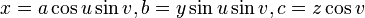$x = a\cos u \sin v, b = y \sin u \sin v, c = z \cos v$

Quantity Meaning in general Value$E,F,G$ for first fundamental form using this parametrization The Riemannian metric is given by$ds^2 = E \, du^2 + 2F \, du \, dv + G \, dv^2$ Fill this in later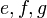$e,f,g$ for second fundamental form using this parametrization Fill this in later Fill this in later
principal curvatures using this parametrization eigenvalues of the shape operator Fill this in later
mean curvature arithmetic mean of the principal curvatures = half the trace of the shape operator Fill this in later
Gaussian curvature product of the principal curvatures = determinant of shape operator Fill this in later

## Verification of theorems

### Gauss-Bonnet theorem

Fill this in later